## Tamilnadu Samacheer Kalvi 12th Maths Solutions Chapter 10 Ordinary Differential Equations Ex 10.8

Question 1.
The rate of increase in the number of bacteria in a certain bacteria culture is proportional to the number present. Given that the number triples in 5 hours, find how many bacteria will be present after 10 hours?
Solution:
Let ‘P’ be the number of bacteria present at time ‘t’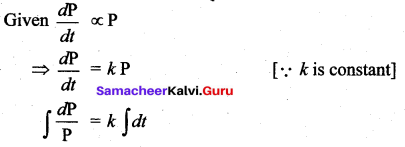log P = kt + c
P = ekt + c
P = ekt c …(1)
Initially, when t = 0, let P = P0
P0 = e° c ⇒ c = P0
Given t = 5, P = 3P0
Substituting in (1)
3 P0 = e5k. P0 ⇒ e5k = 3
Again, when t = 10
P = e10k. c
P = (e5k)2 . P0 = (3)2 P0
P = 9P0
After 10 hours, the number of bacteria is 9 times the original bacteria present.Question 2.
Find the population of a city at any time t, given that the rate of increase of population is proportional to the population at that instant and that in a period of 40 years the population increased from 3,00,000 to 4,00,000.
Solution:
Let the population of a city be ’P’ at time ‘t’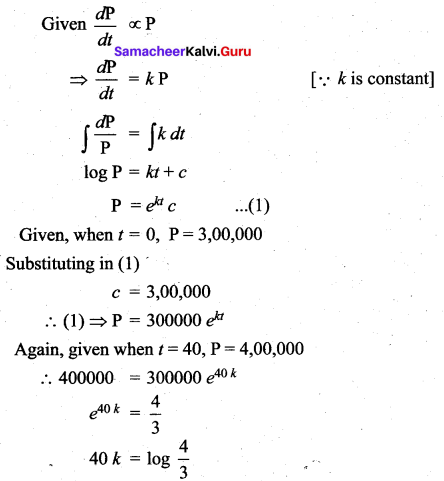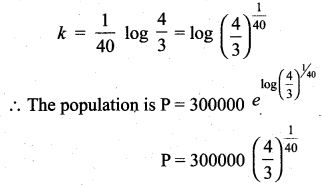Question 3.
The equation of electromotive force for an electric circuit containing resistance and self inductance is E = Ri + L $$\frac{d i}{d t}$$, where E is the electromotive force is given to the circuit, R the resistance and L, the coefficient of induction. Find the current i at time t when E = 0.
Solution:
Given equation of electromotive force is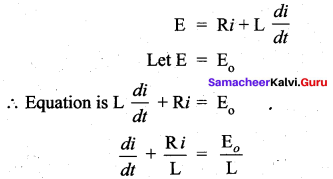This is a Linear differential equation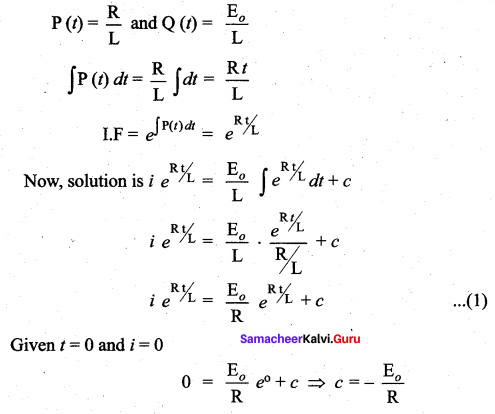Substituting in (1)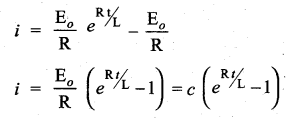Question 4.
The engine of a motor boat moving at 10 m/s is shut off. Given that the retardation at any subsequent time (after shutting off the engine) equal to the velocity at that time. Find the velocity after 2 seconds of switching off the engine.
Solution:
Given, the retardation experienced by a moving motor boat after its engine is shut off is given by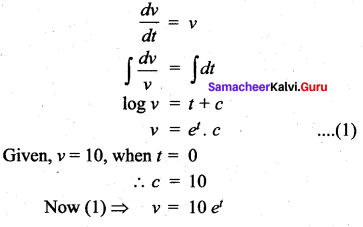Again, given t = 2 ⇒ v = 10 e2

Question 5.
Suppose a person deposits 10,000 Indian rupees in a bank account at the rate of 5% per annum compounded continuously. How much money will be in his bank account 18 months later?
Solution:
Let P be the principal
Rate of interest 5 %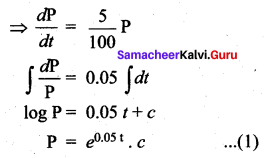Given,when t = 0,P = 10000
P = 10000 e0.05(1.5) = 10000 e0.075Question 6.
Assume that the rate at which radioactive nuclei decay is proportional to the number of such nuclei that are present in a given sample. In a certain sample 10% of the original number of radioactive nuclei have undergone disintegration in a period of 100 years. What percentage of the original radioactive nuclei will remain after 1000 years?
Solution:
Let x (t) denote the number of nuclei present in a sample at anytime ‘t’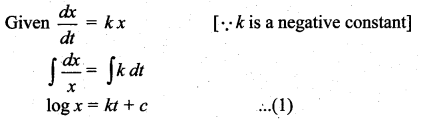Let xo denote the number of nuclei present in the given sample at time t = 0
∴ from (1) ⇒ c = log k0
∴ x = x0ek t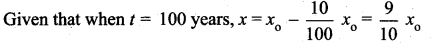∴ Required percentage after 1000 years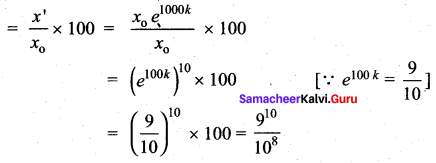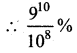of radioactive element will remain after 1000 years.

Question 7.
Water at temperature 100° C cools in 10 minutes to 80° C in a room temperature of 25° C. Find
(i) The temperature of water after 20 minutes
(ii) The time when the temperature is 40° C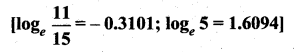Solution: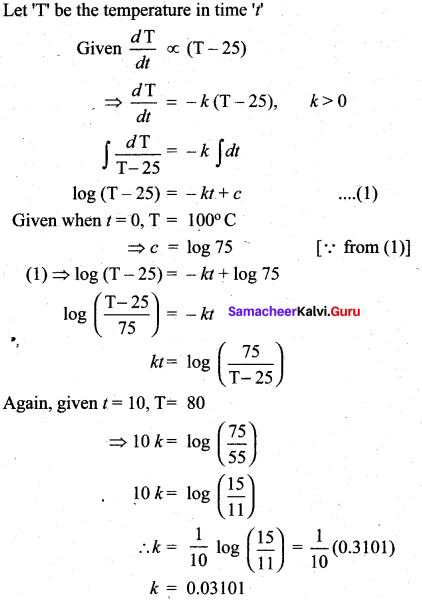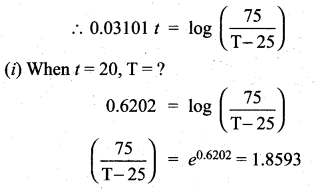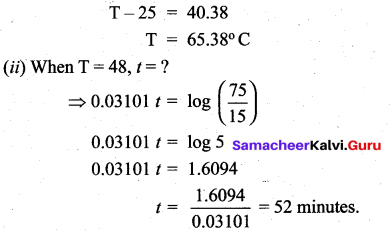Question 8.
At 10.00 A.M. a woman took a cup of hot instant coffee from her microwave oven placed it on a nearby Kitchen counter to cool. At this instant the temperature of the coffee was 180° F, and 10 minutes later it was 160° F . Assume that constant temperature of the kitchen was 70° F.
(i) What was the temperature of the coffee at 10.15 A.M.?
(ii) The woman likes to drink coffee when its temperature is between 130° F and 140° F. between what times should she have drunk the coffee?
Solution:
Change in Temperature is proportional to the difference in temperature (Newton’s Cooling
Law)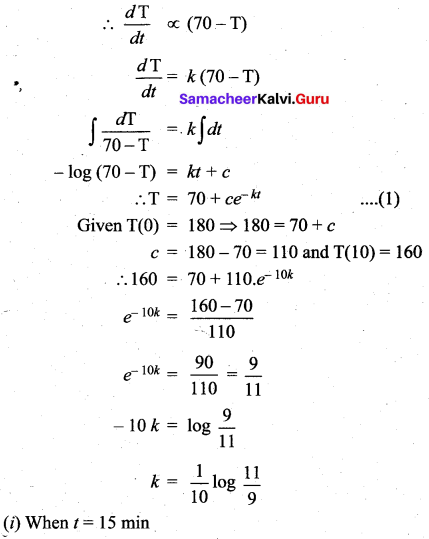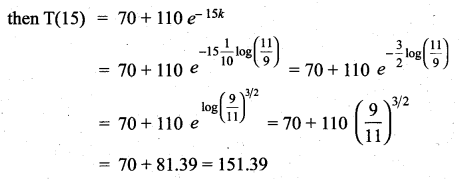(ii) Substituting T = 130 and T = 140
We get t = 22.523

Question 9.
A pot of boiling water at 100° C is removed from a stove at time t = 0 and left to cool in the kitchen. After 5 minutes, the water temperature has decreased to 80° C, and another 5 minutes later it has dropped to 65° C. Determine the temperature of the kitchen.
Solution:
Let A be the temperature of the kitchen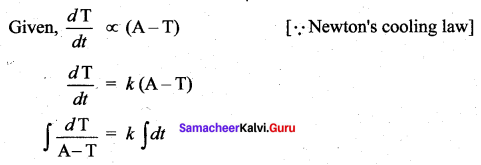log (A – T) = kt + c
⇒ T = A + ce-kt …. (1)
Given, T(0) = 100,
T(5) = 80,
T (10) = 65
Substituting in (1), we get
100 = A+c
80 = A + ce-5k
65 = A + ce -10k
Solving these linear equations,
We get A = 11° (Kitchen Temperature)Question 10.
A tank initially contains 50 liters of pure water. Starting at time t = 0 a brine containing with 2 grams of dissolved salt per litre flows into the tank at the rate of 3 liters per minute. The mixture is kept uniform by stirring and the well-stirred mixture simultaneously flows out of the tank at the same rate. Find the amount of salt present in the tank at any time t > 0 .
Solution:
Let ‘x’ be the amount of salt present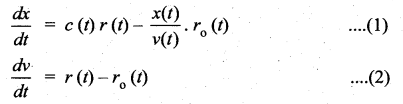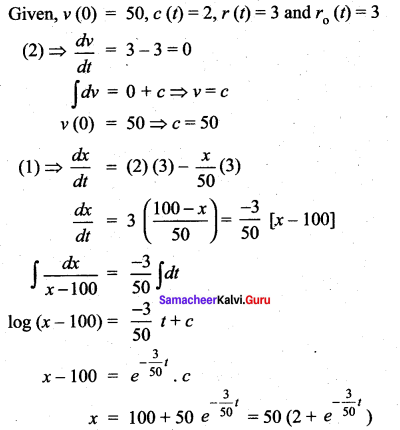Since the tank initially contains only pure water, the initial amount of salt is zero. i.e., x (0) = 0

### Samacheer Kalvi 12th Maths Solutions Chapter 10 Ordinary Differential Equations Ex 10.8 Additional Problems

Question 1.
In a certain chemical reaction the rate of conversion of a substance at time t is proportional to the quantity of the substance still untransformed at that instant. At the end of one hour, 60 grams remain and at the end of 4 hours 21 grams. How many grams of the substance was there initially?
Solution:
Let A be the substance at time t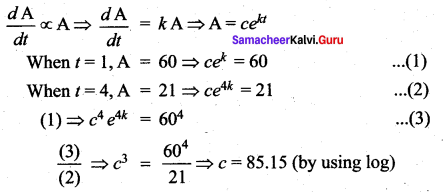Initially i.e., when t = 0, A = c = 85.15 gms (app.)
Hence initially there was 85.15 gms (approximately) of the substance.

Question 2.
The temperature T of a cooling object drops at a rate proportional to the difference T – S, where S is constant temperature of surrounding medium. If initially T = 150° C, find the temperature of the cooling object at any time t.
Solution:
Let T be the temperature of the cooling object at any time t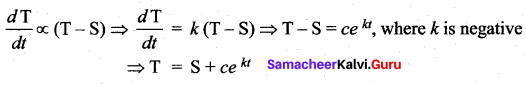when t = 0, T = 150 ⇒ 150 = S + c ⇒ c = 150 – S
∴ The temperature of the cooling object at any time T = S + (150 – S)ekt
Note:
Since k is negative, as t increases T decreases.
It is a decay problem. Instead of k one may take – k where k > 0. Then the answer is
T = S + (150 – S) e-kt.
Again, as t increases T decreases.Question 3.
The sum of Rs. 1000 is compounded continuously, the nominal rate of interest being four per cent per annum. In how many years, will the amount be twice the original principal? [loge 2 = 0.6931]
Solution:
Rate of interest = 4 %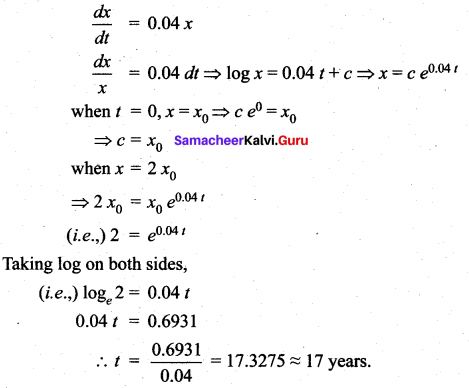Question 4.
A cup of coffee at temperature 100° C is placed in a room whose temperature is 15° C and it cools to 60° C in 5 minutes. Find its temperature after a further interval of 5 minutes.
Solution: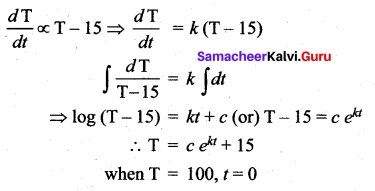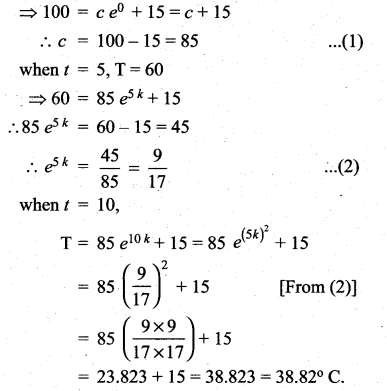Question 5.
The rate at which the population of a city increases at any time is proportional to the population at that time. If there were 1,30,000 people in the city in 1960 and 1,60,000 in 1990, what population may be anticipated in 2020? [loge$$\left(\frac{16}{3}\right)$$ = 0.2070; e-0.42 = 1.52]
Solution: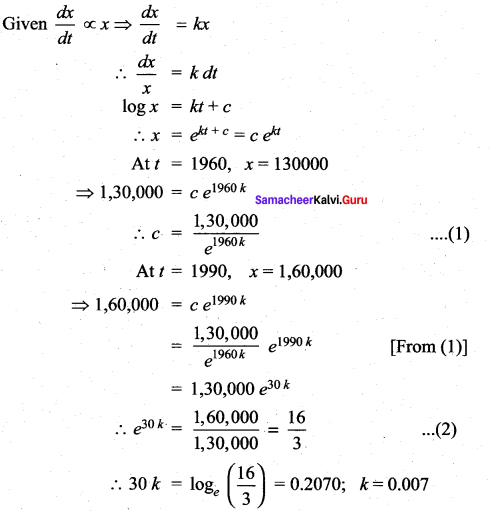∴ P = 1,30,000; e0.007 is the population at any time ‘t’
In the year 2020, (i.e.,) when t = 60
x = 130000 × e0.42 = 1,30,000 × 1.52 = 1,97,600
So, the anticipated population in 2020 will be 1, 97, 600